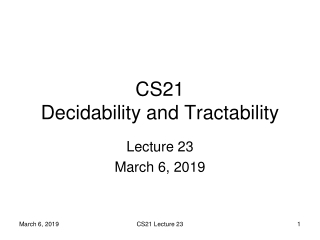# CS21 Decidability and Tractability - PowerPoint PPT PresentationDownload PresentationCS21 Decidability and Tractability

CS21 Decidability and Tractability
Download Presentation## CS21 Decidability and Tractability

- - - - - - - - - - - - - - - - - - - - - - - - - - - E N D - - - - - - - - - - - - - - - - - - - - - - - - - - -
##### Presentation Transcript

1. CS21 Decidability and Tractability Lecture 23 March 6, 2019 CS21 Lecture 23

2. Outline • NP-complete problem: max cut (finishing up) • The complexity class coNP • The complexity class coNP NP • The complexity class PSPACE • a PSPACE-complete problem CS21 Lecture 23

3. MAX CUT Theorem: the following language is NP-complete: MAX CUT = {(G = (V, E), k) : there is a cut S V with at least k edges crossing it} • Proof: • Part 1: MAX CUT NP. Proof? • Part 2: MAX CUT is NP-hard. • reduce from? CS21 Lecture 23

4. MAX CUT is NP-complete • We are reducing from the language: NAE3SAT = {φ : φ is a 3-CNF formula for which there exists a truth assignment in which every clause has at least 1 true literal and at least 1 false literal} to the language: MAX CUT = {(G = (V, E), k) : there is a cut S V with at least k edges crossing it} CS21 Lecture 23

5. MAX CUT is NP-complete • The reduction: • given instance of NAE3SAT (n nodes, m clauses): (x1x2 x3)(x1x4 x5)…(x2x3 x3) • produce graph G = (V, E) with node for each literal x3 x4 x4 • triangle for each 3-clause • parallel edges for each 2-clause x2 x5 x1 x1 x2 x5 x3 CS21 Lecture 23

6. MAX CUT is NP-complete x3 x4 x4 • triangle for each 3-clause • parallel edges for each 2-clause • if cut selects TRUE literals, each clause contributes 2 if NAE, and < 2 otherwise • need to penalize cuts that correspond to inconsistent truth assignments • add ni parallel edges from xi to xi(ni= # occurrences) (repeat variable in 2-clause to make 3-clause for this calculation) x2 x1 x5 x1 x2 x5 x3 CS21 Lecture 23

7. MAX CUT is NP-complete • triangle for each 3-clause • parallel edges for each 2-clause • ni parallel edges from xi to xi • set k = 5m x3 x4 x4 • YES maps to YES • take cut to be TRUE literals in a NAE truth assignment • contribution from clause gadgets: 2m • contribution from (xi, xi) parallel edges: 3m x2 x1 x5 x1 x2 x5 x3 CS21 Lecture 23

8. MAX CUT is NP-complete x3 x4 • triangle for each 3-clause • parallel edges for each 2-clause • ni parallel edges from xi to xi • set k = 5m x4 x2 • NO maps to NO • Claim: if cut has xi, xion same side, then can move one to opposite side without decreasing # edges crossing cut • contribution from (xi, xi) parallel edges: 3m • contribution from clause gadgets must be 2m • conclude: there is a NAE assignment x1 x5 x1 x2 x3 x5 CS21 Lecture 23

9. MAX CUT is NP-complete Claim: if cut has xi, xion same side, then can move one to opposite side without decreasing # edges crossing cut • Proof: xi a + ni a edges ... xi xi ni edges a+b ≤2ni xi ... b + ni ... xi b edges a + ni ≥ a + b or b + ni ≥ a + b xi CS21 Lecture 23

10. coNP • Is NP closed under complement? x L Can we transform this machine: x L x L x L qaccept qreject into a machine with this behavior? qaccept qreject CS21 Lecture 23

11. coNP • language L is in coNP iff its complement (co-L) is in NP • it is believed that NP ≠ coNP • note: P = NP implies NP = coNP • proving NP ≠ coNP would prove P ≠ NP • another major open problem… CS21 Lecture 23

12. coNP • canonical coNP-complete language: UNSAT = {φ : φ is an unsatisfiable 3-CNF formula} • proof? CS21 Lecture 23

13. coNP Disjunctive Normal Form = OR of ANDs • another example 3-DNF-TAUTOLOGY = {φ : φ is a 3-DNF formula and for all x, φ(x) =1} • proof? • another example: EQUIV-CIRCUIT = {(C1, C2) : C1 and C2 are Boolean circuits and for all x, C1(x) = C2(x)} • proof? CS21 Lecture 23

14. Quantifier characterization of coNP CS21 Lecture 23

15. Proof interpretation of coNP “proof” “short” proof “proof verifier” CS21 Lecture 23

16. coNP • what complexity class do the following languages belong in? • COMPOSITES = {x : integer x is a composite} • PRIMES = {x : integer x is a prime number} • GRAPH-NONISOMORPHISM = {(G, H) : G and H are graphs that are not isomorphic} • EXPANSION = {(G = (V,E), > 0): every subset S V of size at most |V|/2 has at least |S| neighbors} CS21 Lecture 23

17. coNP • Picture of the way we believe things are: coNP EXP decidable languages P NP NP coNP CS21 Lecture 23

18. NP coNP • Might guess NP coNP = P by analogy with RE (since RE coRE = DECIDABLE) • Not believed to be true. • A problem in NP coNP not believed to be in P: L = {(x, k): integer x has a prime factor p < k} (decision version of factoring) CS21 Lecture 23

19. NP coNP CS21 Lecture 23

20. PRIMES in NP CS21 Lecture 23

21. Summary • Picture of the way we believe things are: EXP coNP (decision version of ) FACTORING decidable languages P NP NP coNP CS21 Lecture 23